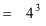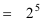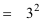# On-Line Scientific Calculator

You need JavaScript switched on your browser for this to work. This mostly appears to work, and has a few quirks you have to get used to. After each calculation, click on the "Clear display" button.

### Mini-Manual for this calculator

Key Meaning Example

√x
find the square root of x √9 = 3
find x squared 6² = 36
find x cubed 2³ = 8
find the natural number, e, raised to the power a e0 = 1
e1 = 2.1718
e2 = 7.389
log
find the base-10 log of a number

log 1 = 0
log 2 = 0.3010
log 10 = 1
log 100 = 2
100 = 102

ln
find the natural log of a number

ln 1 = 0
ln 2 = 0.6931 or
2 = e0.6931

lg
find the base-2 log of a number

lg 1 = 0
lg 2 = 1
lg 3 = 1.0986
lg 4 = 2
4 = 22

sin/cos/tan
Find the sine, cosine or tangent of an angle
asin/acos/atan
Find the arcsine, arccosine, or arctangent of an angle. This is the reverse process of sin/cos/tan.
ran
Generate a random number between 0 and 1 (pseudo random).
rnd
Round the displayed number to the nearest integer.
pow
Raise some number x to the power y. Pressing "pow" clears the display for your next number.

4 pow 3 = 642 pow 5 = 323 pow 2 = 9e
Display the number e (natural number) e = 2.71828
π
Display the number π (Pi) π = 3.14159
STO/RCL
Store a number in memory / Recall a number from memory
M+